## mlcourse.ai – Open Machine Learning Course¶

Author: Dmitriy Sergeyev. Translated by @borowis. This material is subject to the terms and conditions of the Creative Commons CC BY-NC-SA 4.0. Free use is permitted for any non-commercial purpose.

This is a static version of a Jupyter notebook. You can also check out the latest version in the course repository, the corresponding interactive web-based Kaggle Kernel or a video lecture.

# Topic 9. Part 1. Time series analysis in Python¶

Hi there!

We continue our open machine learning course with a new article on time series.

Let's take a look at how to work with time series in Python: what methods and models we can use for prediction, what double and triple exponential smoothing is, what to do if stationarity is not your favorite thing, how to build SARIMA and stay alive, how to make predictions using xgboost... In addition, all of this will be applied to (harsh) real world examples.

# Outline¶

1. Introduction
• Basic definitions
• Quality metrics
2. Move, smoothe, evaluate
• Rolling window estimations
• Exponential smoothing, Holt-Winters model
• Time-series cross validation, parameters selection
3. Econometric approach
• Stationarity, unit root
• Getting rid of non-stationarity
• SARIMA intuition and model building
4. Linear (and not quite) models on time series-models-on-time-series)
• Feature extraction
• Linear models, feature importance
• Regularization, feature selection
• XGBoost
5. Conclusion
6. Demo assignment
7. Useful resources

In my day-to-day, I encounter time-series related tasks almost every day. The most frequent questions asked are the following: what will happen with our metrics in the next day/week/month/etc., how many user will install our app, how much time will they spend online, how many actions will users complete, and so on. We can approach these prediction tasks using different methods depending on the required quality of the prediction, length of the forecast period, and, of course, the time within which we have to choose features and tune parameters to achieve desired results.

# 1. Introduction¶

We begin with a simple definition of time series:

Time series is a series of data points indexed (or listed or graphed) in time order.

Therefore, the data is organized by relatively deterministic timestamps, and may, compared to random sample data, contain additional information that we can extract.

Let's import some libraries. First, we will need the statsmodels library, which has many statistical modeling functions, including time series. For R afficionados who had to move to Python, statsmodels will definitely look more familiar since it supports model definitions like 'Wage ~ Age + Education'.

In :
import warnings                                  # do not disturbe mode
warnings.filterwarnings('ignore')

import numpy as np                               # vectors and matrices
import pandas as pd                              # tables and data manipulations
import matplotlib.pyplot as plt                  # plots
import seaborn as sns                            # more plots

from dateutil.relativedelta import relativedelta # working with dates with style
from scipy.optimize import minimize              # for function minimization

import statsmodels.formula.api as smf            # statistics and econometrics
import statsmodels.tsa.api as smt
import statsmodels.api as sm
import scipy.stats as scs

from itertools import product                    # some useful functions
from tqdm import tqdm_notebook

%matplotlib inline


As an example, let's look at real mobile game data. Specifically, we will look into ads watched per hour and in-game currency spend per day:

In :
ads = pd.read_csv('../input/ads.csv', index_col=['Time'], parse_dates=['Time'])

In :
plt.figure(figsize=(15, 7))
plt.grid(True)
plt.show()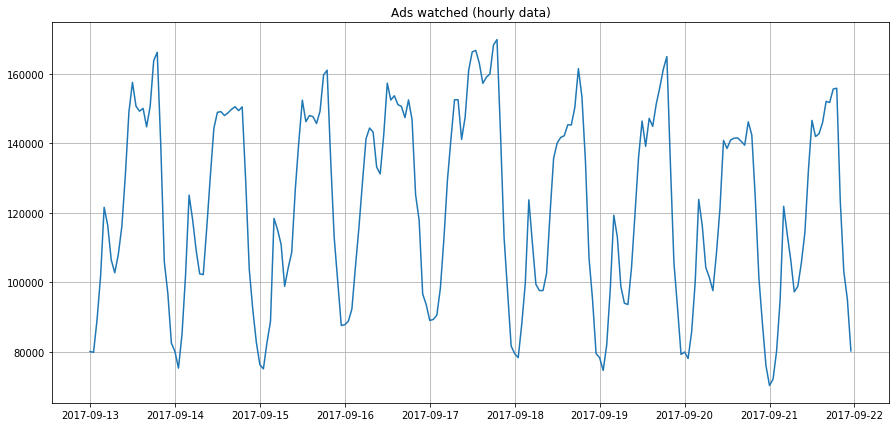In :
plt.figure(figsize=(15, 7))
plt.plot(currency.GEMS_GEMS_SPENT)
plt.title('In-game currency spent (daily data)')
plt.grid(True)
plt.show()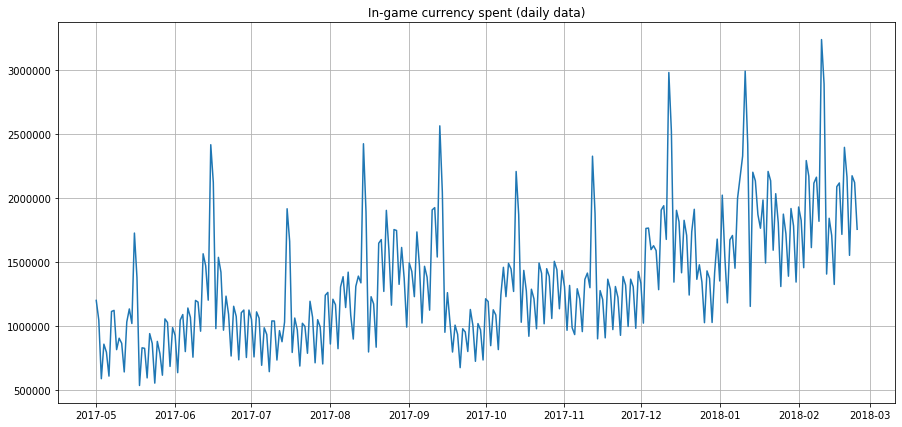## Forecast quality metrics¶

Before we begin forecasting, let's understand how to measure the quality of our predictions and take a look at the most commonly used metrics.

• R squared: coefficient of determination (in econometrics, this can be interpreted as the percentage of variance explained by the model), $(-\infty, 1]$

$R^2 = 1 - \frac{SS_{res} }{SS_{tot} }$

sklearn.metrics.r2_score


• Mean Absolute Error: this is an interpretable metric because it has the same unit of measurment as the initial series, $[0, +\infty)$

$MAE = \frac{\sum\limits_{i=1}^{n} |y_i - \hat{y}_i|}{n}$

sklearn.metrics.mean_absolute_error


• Median Absolute Error: again, an interpretable metric that is particularly interesting because it is robust to outliers, $[0, +\infty)$

$MedAE = median(|y_1 - \hat{y}_1|, ... , |y_n - \hat{y}_n|)$

sklearn.metrics.median_absolute_error


• Mean Squared Error: the most commonly used metric that gives a higher penalty to large errors and vice versa, $[0, +\infty)$

$MSE = \frac{1}{n}\sum\limits_{i=1}^{n} (y_i - \hat{y}_i)^2$

sklearn.metrics.mean_squared_error


• Mean Squared Logarithmic Error: practically, this is the same as MSE, but we take the logarithm of the series. As a result, we give more weight to small mistakes as well. This is usually used when the data has exponential trends, $[0, +\infty)$

$MSLE = \frac{1}{n}\sum\limits_{i=1}^{n} (log(1+y_i) - log(1+\hat{y}_i))^2$

sklearn.metrics.mean_squared_log_error


• Mean Absolute Percentage Error: this is the same as MAE but is computed as a percentage, which is very convenient when you want to explain the quality of the model to management, $[0, +\infty)$

$MAPE = \frac{100}{n}\sum\limits_{i=1}^{n} \frac{|y_i - \hat{y}_i|}{y_i}$

def mean_absolute_percentage_error(y_true, y_pred):
return np.mean(np.abs((y_true - y_pred) / y_true)) * 100

In :
# Importing everything from above

from sklearn.metrics import r2_score, median_absolute_error, mean_absolute_error
from sklearn.metrics import median_absolute_error, mean_squared_error, mean_squared_log_error

def mean_absolute_percentage_error(y_true, y_pred):
return np.mean(np.abs((y_true - y_pred) / y_true)) * 100


Now that we know how to measure the quality of the forecasts, let's see what metrics we can use and how to translate the results for the boss. After that, one small detail remains - building the model.

# 2. Move, smoothe, evaluate¶

Let's start with a naive hypothesis: "tomorrow will be the same as today". However, instead of a model like $\hat{y}_{t} = y_{t-1}$ (which is actually a great baseline for any time series prediction problems and sometimes is impossible to beat), we will assume that the future value of our variable depends on the average of its $k$ previous values. Therefore, we will use the moving average.

$\hat{y}_{t} = \frac{1}{k} \displaystyle\sum^{k}_{n=1} y_{t-n}$

In :
def moving_average(series, n):
"""
Calculate average of last n observations
"""
return np.average(series[-n:])

moving_average(ads, 24) # prediction for the last observed day (past 24 hours)

Out:
116805.0

Unfortunately, we cannot make predictions far in the future -- in order to get the value for the next step, we need the previous values to be actually observed. But moving average has another use case - smoothing the original time series to identify trends. Pandas has an implementation available with DataFrame.rolling(window).mean(). The wider the window, the smoother the trend. In the case of very noisy data, which is often encountered in finance, this procedure can help detect common patterns.

In :
def plotMovingAverage(series, window, plot_intervals=False, scale=1.96, plot_anomalies=False):

"""
series - dataframe with timeseries
window - rolling window size
plot_intervals - show confidence intervals
plot_anomalies - show anomalies

"""
rolling_mean = series.rolling(window=window).mean()

plt.figure(figsize=(15,5))
plt.title("Moving average\n window size = {}".format(window))
plt.plot(rolling_mean, "g", label="Rolling mean trend")

# Plot confidence intervals for smoothed values
if plot_intervals:
mae = mean_absolute_error(series[window:], rolling_mean[window:])
deviation = np.std(series[window:] - rolling_mean[window:])
lower_bond = rolling_mean - (mae + scale * deviation)
upper_bond = rolling_mean + (mae + scale * deviation)
plt.plot(upper_bond, "r--", label="Upper Bond / Lower Bond")
plt.plot(lower_bond, "r--")

# Having the intervals, find abnormal values
if plot_anomalies:
anomalies = pd.DataFrame(index=series.index, columns=series.columns)
anomalies[series<lower_bond] = series[series<lower_bond]
anomalies[series>upper_bond] = series[series>upper_bond]
plt.plot(anomalies, "ro", markersize=10)

plt.plot(series[window:], label="Actual values")
plt.legend(loc="upper left")
plt.grid(True)


Let's smooth by the previous 4 hours.

In :
plotMovingAverage(ads, 4)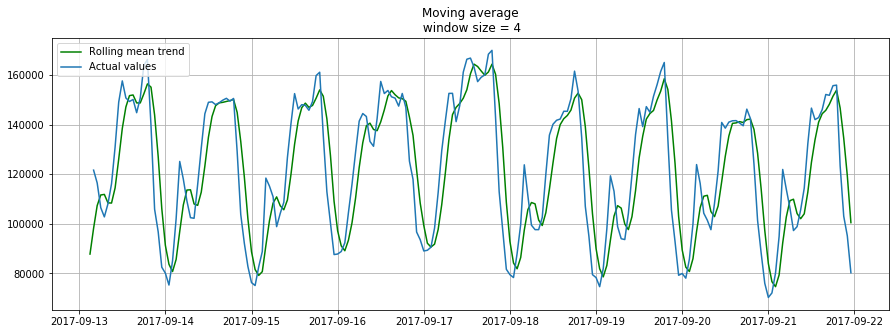Now let's try smoothing by the previous 12 hours.

In :
plotMovingAverage(ads, 12)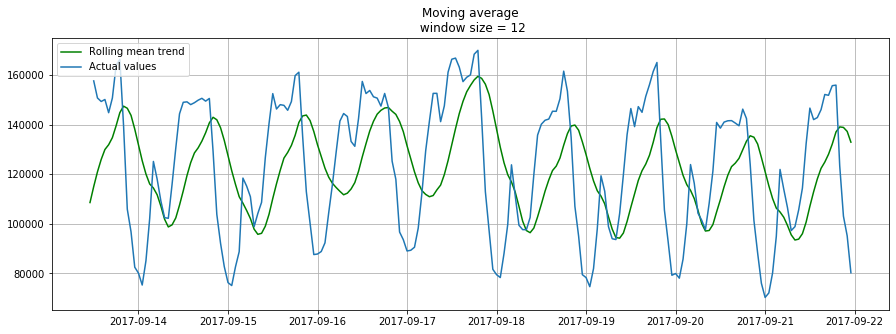Now with the previous 24 hours, we get the daily trend.

In :
plotMovingAverage(ads, 24)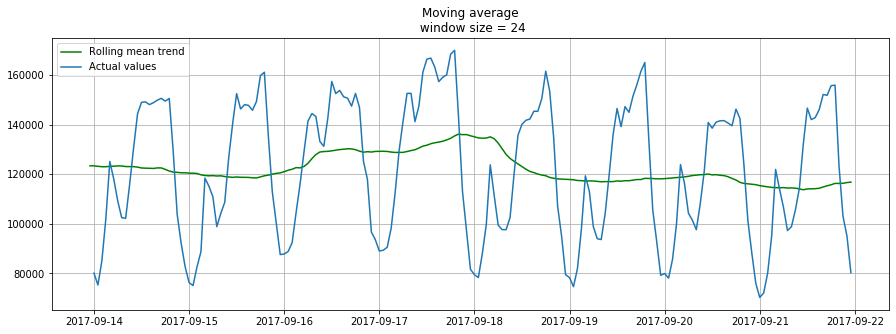When we applied daily smoothing on hourly data, we could clearly see the dynamics of ads watched. During the weekends, the values are higher (more time to play on the weekends) while fewer ads are watched on weekdays.

We can also plot confidence intervals for our smoothed values.

In :
plotMovingAverage(ads, 4, plot_intervals=True)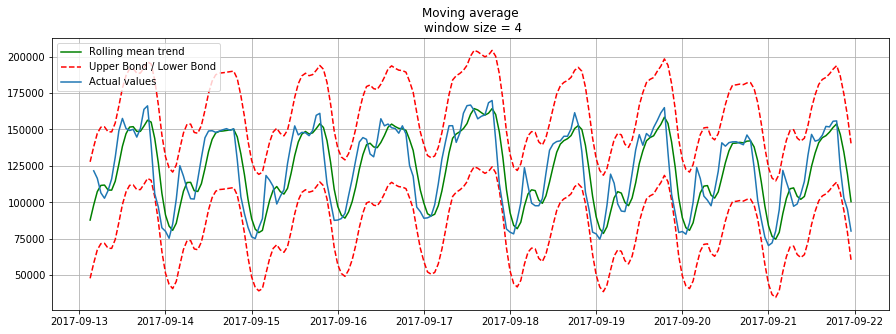Now, let's create a simple anomaly detection system with the help of moving average. Unfortunately, in this particular dataset, everything is more or less normal, so we will intentionally make one of the values abnormal in our dataframe ads_anomaly.

In :
ads_anomaly = ads.copy()


Let's see if this simple method can catch the anomaly.

In :
plotMovingAverage(ads_anomaly, 4, plot_intervals=True, plot_anomalies=True)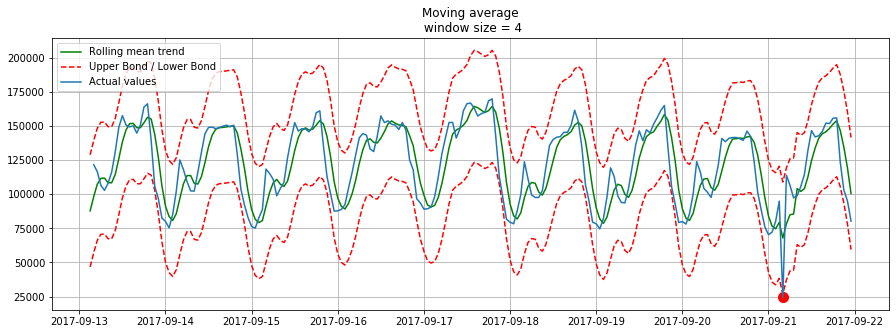Neat! What about the second series?

In :
plotMovingAverage(currency, 7, plot_intervals=True, plot_anomalies=True) # weekly smoothing